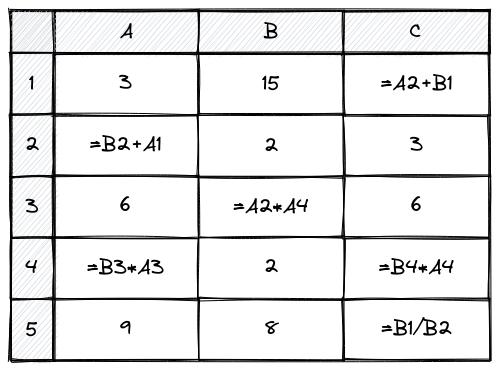hjr265Cefalo SUST Inter Univers...
Limits 2.5s, 512 MB

Are you trying to find a problem that is easy to solve? Even if it involves writing a lot of code? This is the problem you have been looking for!

In this problem, you need to build a tiny spreadsheet application.

You will be given the initial contents of the spreadsheet. For each cell in the spreadsheet, you will be given either a value or a formula. All values are integer numbers. All formulae start with an equal (=) sign, followed by the expression.

Here is what a spreadsheet may look like:All formulae in the spreadsheet will be of the following form: {CELL1}{OP}{CELL2}. Here, {CELL1} and {CELL2} are references to specific cells (can be the same cell), and {OP} is an operator (one of + addition, - subtraction, * multiplication, / integer division).

Cell references are always of the form {COL}{ROW} (e.g. B2, B is the column alphabet, 2 is the row number). While rows are identified by a number (1-based), columns are identified by one or more alphabets. Column labels go from “A” to “Z”, “AA” to “AZ”, “BA” to “BZ”, “CA”, to “CZ”, …, “ZA” to “ZZ”, “AAA” to “AAZ”, and so on.

You will have to print the entire spreadsheet with the final value of every cell. If the cell contains a value, print it. If the cell contains a formula, evaluate the expression and print the final value.

Final values (after evaluation of expression) should always be one of the following:

• An integer: When the expression can be evaluated.
• "CYCLE": When there is a cycle in the cells referenced in the expression (e.g. cell B3 references A4, which contains a formula that references B3 again).
• "DIVZERO": When there is a division by 0 during the evaluation of an expression.
• “OVERFLOW”: When any operation causes the value of the field to overflow or underflow a 32-bit signed integer. The minimum value a 32-bit signed integer can hold is -2147483648 and the maximum is 2147483647.

When evaluating the formula in any cell, it may happen that through references in that formula you have to jump to different cells, and then eventually arrive back at the cell where you started from. This is a cycle. And all the cells in this cycle should shown “CYCLE”.

Any cell that references itself will be result in a “CYCLE”. Any cell that references a cell in a cycle will also show “CYCLE”.

In case the formula in a cell references another cell with either “DIVZERO” or “OVERFLOW”, then the formula will evaluate to an error. In this case the error on the left-hand side of the formula will have higher priority. For example, =DIVZERO+OVERFLOW will evaluate to “DIVZERO”. And =OVERFLOW+DIVZERO will evaluate to “OVERFLOW”.

If the left-hand side of a formula is an integer or a valid reference, but the right-hand side is an error, the cell will show the same error.

## Input

The input will start with $R$ ($1 \le R \le 1000$) and $C$ ($1 \le C \le 1000$), the number of rows and columns in the spreadsheet.

The following $R$ lines each will contain $C$ space-separated tokens. Each token is either a 32-bit signed integer or a string prefixed with “=” (w/o quotes).

Tokens prefixed with “=” will have the format {CELL1}{OP}{CELL2}. {CELL1} and {CELL2} will contain one or more uppercase alphabets followed by an integer. {OP} will be one of the following {+, -, *, /}.

## Output

Print $R$ lines with $C$ tokens in each (after evaluating all expressions in the given spreadsheet). The tokens must be either integer or one of “CYCLE”, “DIVZERO” and “OVERFLOW” (w/o quotes).

## Samples

InputOutput
3 3
1 2 3
4 =A1+C1 5
=A1+A2 =B1+B2 =C1+C2

1 2 3
4 4 5
5 6 8

InputOutput
3 3
1 =A1+C1 1
=A1+B1 =C2+A1 =A2+C3
1 2 =A3+B2

1 2 1
3 CYCLE CYCLE
1 2 CYCLE

InputOutput
3 3
0 1 2
2 =A2/A1 1
2 1 0

0 1 2
2 DIVZERO 1
2 1 0### Submit